# Orthogonal projector

(diff) ← Older revision | Latest revision (diff) | Newer revision → (diff)
A mappingof a Hilbert spaceonto a subspaceof it such that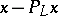is orthogonal to: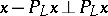. An orthogonal projector is a bounded self-adjoint operator, acting on a Hilbert space, such thatand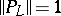. On the other hand, if a bounded self-adjoint operator acting on a Hilbert spacesuch that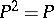is given, thenis a subspace, andis an orthogonal projector onto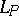. Two orthogonal projectorsare called orthogonal if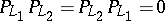; this is equivalent to the condition that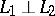.
Properties of an orthogonal projector. 1) In order that the sum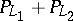of two orthogonal projectors is itself an orthogonal projector, it is necessary and sufficient that, in this case; 2) in order that the composite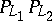is an orthogonal projector, it is necessary and sufficient that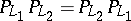, in this case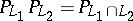.
An orthogonal projector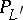is called a part of an orthogonal projector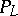if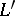is a subspace of. Under this condition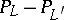is an orthogonal projector on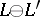— the orthogonal complement toin. In particular,is an orthogonal projector on.